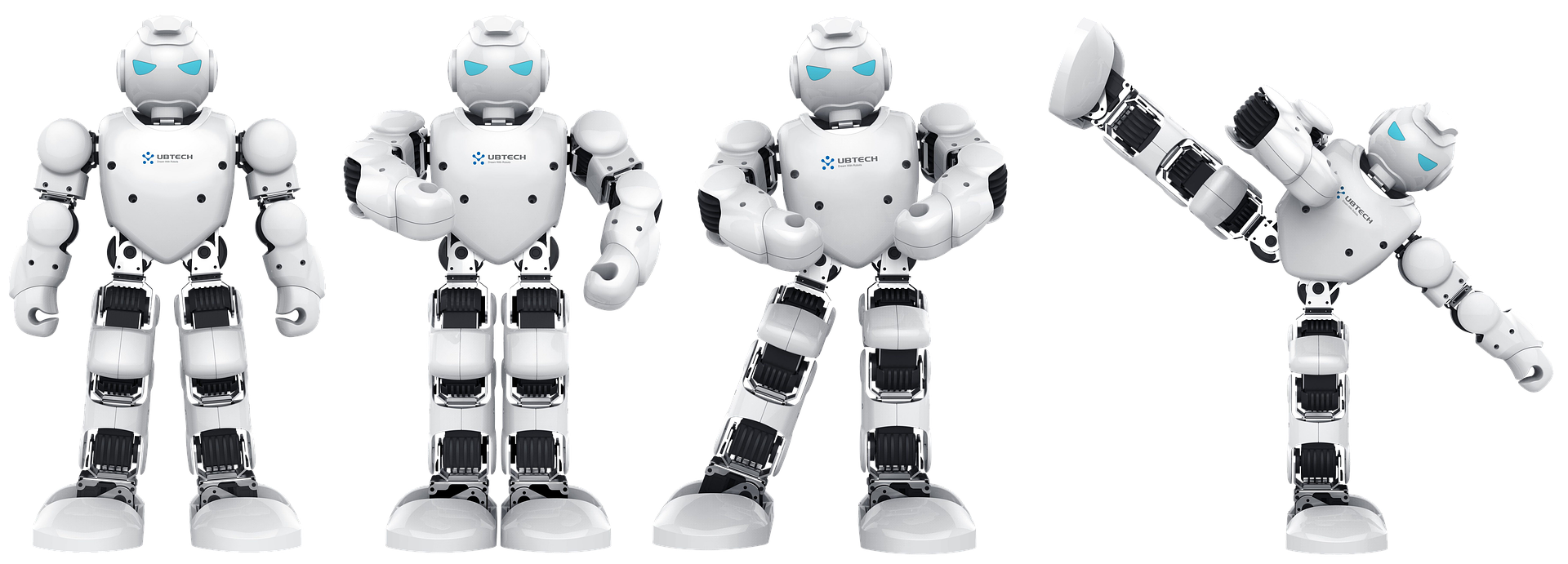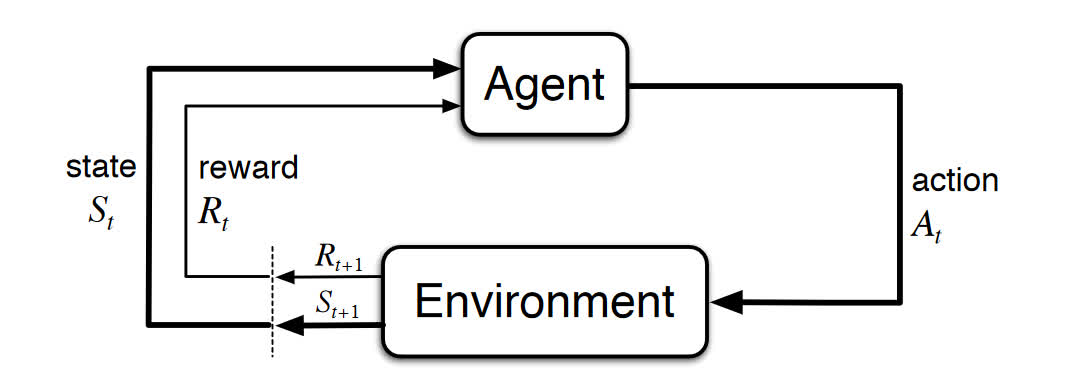# Reinforcement Learning - Goal Oriented Intelligence

Deep Learning Course 4 of 5 - Level: Advanced

## Markov Decision Processes (MDPs) - Structuring a Reinforcement Learning Problem

### video

expand_more chevron_left

### text

expand_more chevron_left

### Markov Decision Processes (MDPs) - Structuring a Reinforcement Learning Problem

What's up, guys? In this post, we're going to discuss Markov decision processes, or MDPs. This topic will lay the bedrock for our understanding of reinforcement learning, so let's get to it!

### Components of an MDP

Markov decision processes give us a way to formalize sequential decision making. This formalization is the basis for structuring problems that are solved with reinforcement learning.To kick things off, let's discuss the components involved in an MDP.

In an MDP, we have a decision maker, called an agent, that interacts with the environment it's placed in. These interactions occur sequentially over time. At each time step, the agent will get some representation of the environment's state. Given this representation, the agent selects an action to take. The environment is then transitioned into a new state, and the agent is given a reward as a consequence of the previous action.

Components of an MDP:
• Agent
• Environment
• State
• Action
• Reward

This process of selecting an action from a given state, transitioning to a new state, and receiving a reward happens sequentially over and over again, which creates something called a trajectory that shows the sequence of states, actions, and rewards.

Throughout this process, it is the agent's goal to maximize the total amount of rewards that it receives from taking actions in given states. This means that the agent wants to maximize not just the immediate reward, but the cumulative rewards it receives over time.

It is the agent's goal to maximize the cumulative rewards.

Alright, let's get a bit mathy and represent an MDP with mathematical notation. This will make things easier for us going forward.

### MDP notation

We're now going to repeat what we just casually discussed but in a more formal and mathematically notated way.

In an MDP, we have a set of states $$\boldsymbol{S}$$, a set of actions $$\boldsymbol{A}$$, and a set of rewards $$\boldsymbol{R}$$. We'll assume that each of these sets has a finite number of elements.

At each time step $$t = 0,1,2,\cdots$$, the agent receives some representation of the environment's state $$S_t \in \boldsymbol{S}$$. Based on this state, the agent selects an action $$A_t \in \boldsymbol{A}$$. This gives us the state-action pair $$(S_t,A_t)$$.

Time is then incremented to the next time step $$t+1$$, and the environment is transitioned to a new state $$S_{t+1} \in \boldsymbol{S}$$. At this time, the agent receives a numerical reward $$R_{t+1} \in \boldsymbol{R}$$ for the action $$A_t$$ taken from state $$S_t$$.

We can think of the process of receiving a reward as an arbitrary function $$f$$ that maps state-action pairs to rewards. At each time $$t$$, we have $$f(S_{t}, A_{t}) = R_{t+1}\text{.}$$

The trajectory representing the sequential process of selecting an action from a state, transitioning to a new state, and receiving a reward can be represented as $$S_0,A_0,R_1,S_1,A_1,R_2,S_2,A_2,R_3,\cdots$$

This diagram nicely illustrates this entire idea.Let's break down this diagram into steps.

1. At time $$t$$, the environment is in state $$S_t$$.
2. The agent observes the current state and selects action $$A_t$$.
3. The environment transitions to state $$S_{t+1}$$ and grants the agent reward $$R_{t+1}$$.
4. This process then starts over for the next time step, $$t+1$$.
• Note, $$t+1$$ is no longer in the future, but is now the present. When we cross the dotted line on the bottom left, the diagram shows $$t+1$$ transforming into the current time step $$t$$ so that $$S_{t+1}$$ and $$R_{t+1}$$ are now $$S_t$$ and $$R_t$$.

#### Transition probabilities

Since the sets $$\boldsymbol{S}$$ and $$\boldsymbol{R}$$ are finite, the random variables $$R_t$$ and $$S_t$$ have well defined probability distributions. In other words, all the possible values that can be assigned to $$R_t$$ and $$S_t$$ have some associated probability. These distributions depend on the preceding state and action that occurred in the previous time step $$t-1$$.

For example, suppose $$s' \in \boldsymbol{S}$$ and $$r \in \boldsymbol{R}$$. Then there is some probability that $$S_t=s'$$ and $$R_t=r.$$ This probability is determined by the particular values of the preceding state $$s \in \boldsymbol{S}$$ and action $$a \in \boldsymbol{A}(s)$$. Note that $$\boldsymbol{A}(s)$$ is the set of actions that can be taken from state $$s$$.

Let's define this probability.

For all $$s^{\prime } \in \boldsymbol{S}$$, $$s \in \boldsymbol{S}$$, $$r\in \boldsymbol{R}$$, and $$a\in \boldsymbol{A}(s)$$, we define the probability of the transition to state $$s^{\prime }$$ with reward $$r$$ from taking action $$a$$ in state $$s$$ as:

\begin{equation*} p\left( s^{\prime },r\mid s,a\right) =\Pr \left\{ S_{t}=s^{\prime },R_{t}=r\mid S_{t-1}=s,A_{t-1}=a\right\} \text{.} \end{equation*}

#### Wrapping up

Alright, we now have a formal way to model sequential decision making. How do you feel about Markov decision processes so far? Some of this may take a bit of time to sink in, but if you can understand the relationship between the agent and the environment and how they interact with each other, then you're off to a great start!Like we discussed earlier, MDPs are the bedrock for reinforcement learning, so make sure to get comfortable with what we covered here, and next time we'll build on concept of cumulative rewards. I'll see ya there!

### quiz

expand_more chevron_leftDEEPLIZARD Message notifications

### resources

expand_more chevron_left
Welcome back to this series on reinforcement learning! In this video, we'll discuss Markov decision processes, or MDPs. Markov decision processes give us a way to formalize sequential decision making. This formalization is the basis for structuring problems that are solved with reinforcement learning. We will detail the components that make up an MDP, including: the environment, the agent, the states of the environment, the actions the agent can take in the environment, and the rewards that may be given to the agent for its actions. Sources: Reinforcement Learning: An Introduction, Second Edition by Richard S. Sutton and Andrew G. Bartow http://incompleteideas.net/book/RLbook2020.pdf Playing Atari with Deep Reinforcement Learning by Deep Mind Technologies https://www.cs.toronto.edu/~vmnih/docs/dqn.pdf 🕒🦎 VIDEO SECTIONS 🦎🕒 00:00 Welcome to DEEPLIZARD - Go to deeplizard.com for learning resources 00:30 Help deeplizard add video timestamps - See example in the description 06:04 Collective Intelligence and the DEEPLIZARD HIVEMIND 💥🦎 DEEPLIZARD COMMUNITY RESOURCES 🦎💥 👋 Hey, we're Chris and Mandy, the creators of deeplizard! 👀 CHECK OUT OUR VLOG: 🔗 https://youtube.com/deeplizardvlog 👉 Check out the blog post and other resources for this video: 🔗 https://deeplizard.com/learn/video/my207WNoeyA 💻 DOWNLOAD ACCESS TO CODE FILES 🤖 Available for members of the deeplizard hivemind: 🔗 https://deeplizard.com/resources 🧠 Support collective intelligence, join the deeplizard hivemind: 🔗 https://deeplizard.com/hivemind 🤜 Support collective intelligence, create a quiz question for this video: 🔗 https://deeplizard.com/create-quiz-question 🚀 Boost collective intelligence by sharing this video on social media! ❤️🦎 Special thanks to the following polymaths of the deeplizard hivemind: Tammy Prash Zach Wimpee 👀 Follow deeplizard: Our vlog: https://youtube.com/deeplizardvlog Facebook: https://facebook.com/deeplizard Instagram: https://instagram.com/deeplizard Twitter: https://twitter.com/deeplizard Patreon: https://patreon.com/deeplizard YouTube: https://youtube.com/deeplizard 🎓 Deep Learning with deeplizard: Fundamental Concepts - https://deeplizard.com/learn/video/gZmobeGL0Yg Beginner Code - https://deeplizard.com/learn/video/RznKVRTFkBY Intermediate Code - https://deeplizard.com/learn/video/v5cngxo4mIg Advanced Deep RL - https://deeplizard.com/learn/video/nyjbcRQ-uQ8 🎓 Other Courses: Data Science - https://deeplizard.com/learn/video/d11chG7Z-xk Trading - https://deeplizard.com/learn/video/ZpfCK_uHL9Y 🛒 Check out products deeplizard recommends on Amazon: 🔗 https://amazon.com/shop/deeplizard 📕 Get a FREE 30-day Audible trial and 2 FREE audio books using deeplizard's link: 🔗 https://amzn.to/2yoqWRn 🎵 deeplizard uses music by Kevin MacLeod 🔗 https://youtube.com/channel/UCSZXFhRIx6b0dFX3xS8L1yQ 🔗 http://incompetech.com/ ❤️ Please use the knowledge gained from deeplizard content for good, not evil.

expand_more chevron_leftDEEPLIZARD Message notifications